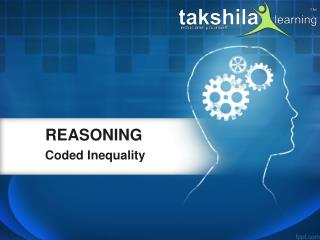DownloadDownload PresentationCoded Inequality

# Coded Inequality

Télécharger la présentation## Coded Inequality

- - - - - - - - - - - - - - - - - - - - - - - - - - - E N D - - - - - - - - - - - - - - - - - - - - - - - - - - -
##### Presentation Transcript

1. REASONING Coded Inequality

2. Coded Inequality • Inequality is one of the most important topics from which 3-5 questions are expected in the bank exams. Inequalities questions are either asked directly or in the coded form & both of the types are important. Here in this ppt, we are discussing the concept of coded inequality:

3. Coded Inequality • Directions (1-5): The symbols @, #, %, \$ and © are used in the following questions with the meaning as mentioned below- ‘P is not smaller than Q’ is represented by ‘P\$Q’ ‘P is not greater than Q’ is represented by ‘P@Q’ ‘P is neither greater than nor equal to Q’ is represented by ‘P#Q’ ‘P is neither equal to nor smaller than Q’ is represented by ‘P©Q’ ‘P is neither smaller than nor greater than Q’ is represented by ‘P%Q’ • Assuming the given statement to be true, find which among the following conclusions is/are definitely true?

4. Coded Inequality Q1. Statements: M © S @ Z % R \$ N Conclusions: I. M © Z     II. R \$ M III.S © N (a) None is true (b) Only I is true (c) Only III is true (d) Either I or II is true (e) All are true S1. Ans (d) To solve coded inequality based questions, we should first convert coded inequality into simplified form: M > S ≤ Z = R ≥ N M © Z i.e. M > Z (false) R \$ M i.e. R ≥ M (false) S © N i.e. S > N (false)

5. Coded Inequality Q2. Statements: A \$ B, B % M, K © M Conclusions: I. K © B     II. M @ A III. A © K (a) Only I and III are true (b) Only II and III are true (c) Only I and II are true (d) All are true (e) None of these S2. Ans (c) On converting coded inequality into simplified form, we get: A ≥ B = M < K K © B i.e. K > B (true)   M @ A i.e. M ≤ A (true) A © K i.e. A > K (false)

6. Coded Inequality Q3. Statements: R \$ M, M © Z, Z @ A, Y © Z Conclusions: I. R © Y     II. Z # R    III.Y © R (a) None is true (b) Only II is true (c) Only I and II are true (d) Only II and III are true (e) All are true S3. Ans (b) On converting coded inequality into simplified form, we get: R ≥ M > Z ≤ A Y > Z R © Y i.e. R > Y (false) Z # R i.e. Z < R (true)   Y © R i.e. Y > R (false)

7. Coded Inequality Q4. Statements: M © S @ Z % R \$ N Conclusions: I. N # Z     II. R # M III. S % N (a) None follows (b) Only I is true (c) Only III is true (d) Either I or II is true (e) All are true S4. Ans (a) On converting coded inequality into simplified form, we get: M > S ≤ Z = R ≥ N N # Z i.e. N < Z (false)  R # M i.e. R < M (false) S % N i.e. S = N (false)

8. Coded Inequality Q5. Statements: R \$ M, M © Z, Z @ A, Y © Z Conclusions: I. R © Z     II. Z # A III.R % A (a) Only I is true (b) Only II is true (c) Only I and II are true (d) Only II and III are true (e) All are true S5. Ans (a) On converting coded inequality into simplified form, we get: R ≥ M > Z ≤ A Y > Z R © Z i.e. R > Z (true)  Z # A i.e. Z < A (false) R % A i.e. R = A (false)